# Solving an integral equation via $$\mathscr{C}^{\star}$$-algebra-valued partial b-metrics

## Abstract

In this paper, we prove some common coupled fixed-point theorems on complete $$\mathscr{C}^{\star}$$-algebra-valued partial b-metric spaces. Some of the well-known facts in the literature are generalized and expanded by the results shown. An example to illustrate our findings is presented. We also explore some of the applications of our key results.

## Introduction

Fixed point theory, one of the active research areas in mathematics, focuses on maps and abstract spaces, see , and the references therein. The notion of coupled fixed points was introduced by Guo and Lakshmikantham . In 2006, Bhaskar and Lakshmikantham  introduced the concept of a mixed monotonicity property for the first time and investigated some coupled fixed point theorems for such mappings. As a result, many authors obtained many coupled fixed point and coupled coincidence point theorems, see  and the references therein. In 2014, Ma et al.  initially introduced the concept of $$\mathscr{C}^{\star}$$-algebra-valued metric spaces, and proved some fixed point theorems for self-maps with contractive or expansive conditions on such spaces. In 2019, Chandok et al.  proved some fixed point theorems on $$C^{*}$$-algebra-valued partial metric spaces. In 2021, Mlaiki et al.  proved some fixed point theorems on $$C^{*}$$-algebra-valued partial b-metric spaces. In this paper, we prove some coupled fixed point theorems on $$C^{*}$$-algebra-valued partial b-metric spaces.

## Preliminaries

First of all, we recall some basic definitions, notations, and results of $$\mathscr{C}^{\star}$$-algebra that can be found in . Let $$\mathscr{H}$$ be a unital algebra. An involution on $$\mathscr{H}$$ is a conjugate-linear map $$r \rightarrow r^{\star}$$ on $$\mathscr{H}$$ such that $$r^{\star \star} = r$$ and $$(rs)^{\star}= s^{\star}r^{\star}$$ for any $$r, s \in \mathscr{H}$$. The pair $$(\mathscr{H},\star )$$ is called a -algebra. A -algebra $$\mathscr{H}$$ together with a complete submultiplicative norm such that $$\Vert r^{\star}\Vert = \Vert r \Vert$$, is said to be a Banach -algebra. Furthermore, a $$\mathscr{C}^{\star}$$-algebra is a Banach -algebra with $$\Vert r^{\star} r\Vert =\Vert r \Vert ^{2}$$, for all $$r \in \mathscr{H}$$. An element r in $$\mathscr{H}$$ is self-adjoint, or hermitian, if $$r = r^{*}$$. Let $$\mathscr{H}_{sa}$$ be the set of all self-adjoint elements in $$\mathscr{H}$$, and define the spectrum of $$r\in \mathscr{H}$$ to be the set $$\sigma (r) = \{\lambda \in C: \lambda I - r\ {\mathit{is\ not\ invertible}}\}$$. An element r of a $$\mathscr{C}^{\star}$$-algebra $$\mathscr{H}^{\star}$$ is positive if r is hermitian and $$\sigma (r) \subseteq [0,+\infty )$$, where $$\sigma (r)$$ is the spectrum of r. We write $$0_{\mathscr{H}} \preceq r$$ to show that an element r is positive, and denote by $$\mathscr{H}_{+}$$$$and$$$$\mathscr{H}_{\eth}$$ the set of positive elements and the hermitian elements of $$\mathscr{H}$$, respectively, where $$0_{\mathscr{H}}$$ is the zero element in $$\mathscr{H}$$. There is a natural partial ordering on $$\mathscr{H}_{\eth}$$ which is given by $$r \preceq s$$ if and only if $$0_{\mathscr{H}} \preceq s-r$$. It is clear that if $$r, s \in \mathscr{H}_{sa}$$ and $$c\in \mathscr{H}$$, then $$r\preceq s\Rightarrow c^{*}r c\preceq c^{*}s c$$, and that if $$r, s\in \mathscr{H}_{+}$$ are invertible, then $$r\preceq s\Longrightarrow \theta \preceq s^{-1}\preceq r^{-1}$$. From now on, $$\mathscr{H}'$$ will denote the set $$\{r \in \mathscr{H}: rs = sr, \forall s \in \mathscr{H}\}$$.

### Definition 2.1

([7, 24])

Let ϒ be a nonempty set. Suppose that the mapping $$\rho: \Upsilon \times \Upsilon \to \mathscr{H}$$ is defined, with the following properties:

1. 1.

$$0_{\mathscr{H}} \preceq \rho (\aleph,\varpi )$$ for all $$\aleph,\varpi \in \Upsilon$$ and $$\rho (\aleph,\varpi )= 0_{\mathscr{H}}$$ if and only if $$\aleph = \varpi$$;

2. 2.

$$\rho (\aleph,\varpi )= \rho (\varpi,\aleph )$$ for all $$\aleph,\varpi \in \Upsilon$$;

3. 3.

$$\rho (\aleph,\varpi ) \preceq \rho (\aleph,\gamma )+\rho (\gamma, \varpi )$$ for all $$\aleph,\varpi,\gamma \in \Upsilon$$.

Then ρ is said to be a $$\mathscr{C}^{\star}$$-algebra-valued metric on ϒ, and $$(\Upsilon,\mathscr{H}, \rho )$$ is said to be a $$\mathscr{C}^{\star}$$-algebra-valued metric space.

The following definition was introduced by Ma and Jiang .

### Definition 2.2

Let ϒ be a nonempty set and $$s\in \mathscr{H}$$ such that $$s\succeq I$$. Suppose that the mapping $$\rho: \Upsilon \times \Upsilon \to \mathscr{H}$$ is defined, with the following properties:

1. 1.

$$0_{\mathscr{H}} \preceq \rho (\aleph,\varpi )$$ for all $$\aleph,\varpi \in \Upsilon$$;

2. 2.

$$\rho (\aleph,\varpi )= 0_{\mathscr{H}}$$ if and only if $$\aleph = \varpi$$;

3. 3.

$$\rho (\aleph,\varpi )= \rho (\varpi,\aleph )$$ for all $$\aleph,\varpi \in \Upsilon$$;

4. 4.

$$\rho (\aleph,\varpi ) \preceq s(\rho (\aleph,\gamma )+\rho ( \gamma,\varpi ))$$ for all $$\aleph,\varpi,\gamma \in \Upsilon$$.

Then ρ is said to be a $$\mathscr{C}^{\star}$$-algebra-valued b-metric on ϒ, and $$(\Upsilon,\mathscr{H}, \rho )$$ is said to be a $$\mathscr{C}^{\star}$$-algebra-valued b-metric space.

Now, we recall the definition of a $$C^{*}$$-algebra-valued partial b-metric space introduced by Mlaiki et al .

### Definition 2.3

Let ϒ be a nonempty set and $$s\in \mathscr{H}$$ such that $$s\succeq I$$. Suppose that the mapping $$\rho: \Upsilon \times \Upsilon \to \mathscr{H}$$ is defined, with the following properties:

1. (A1)

$$0_{\mathscr{H}} \preceq \rho (\aleph,\varpi )$$ for all $$\aleph,\varpi \in \Upsilon$$ and $$\rho (\aleph,\aleph )=\rho (\varpi,\varpi )= \rho (\aleph,\varpi )$$ if and only if $$\aleph = \varpi$$;

2. (A2)

$$\rho (\aleph,\aleph )\preceq \rho (\aleph,\varpi )$$;

3. (A3)

$$\rho (\aleph,\varpi )= \rho (\varpi,\aleph )$$ for all $$\aleph,\varpi \in \Upsilon$$;

4. (A4)

$$\rho (\aleph,\varpi ) \preceq s(\rho (\aleph,\gamma )+\rho ( \gamma,\varpi ))-\rho (\gamma,\gamma )$$ for all $$\aleph,\varpi,\gamma \in \Upsilon$$.

Then ρ is said to be a $$\mathscr{C}^{\star}$$-algebra-valued partial b-metric on ϒ, and $$(\Upsilon,\mathscr{H}, \rho )$$ is said to be a $$\mathscr{C}^{\star}$$-algebra-valued partial b-metric space.

### Example 2.1

Let $$\Upsilon =[0,1]$$ and $$\mathscr{H}=\mathcal{M}_{2}(\mathbb{C})$$, the class of bounded and linear operators on a Hilbert space $$\mathbb{C}^{2}$$. Define $$\rho: \Upsilon \times \Upsilon \to \mathscr{H}$$ by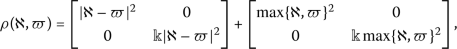where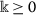and for all $$\aleph,\varpi \in \Upsilon$$. Then, $$(\Upsilon,\mathscr{H},\rho )$$ is a $$\mathscr{C}^{\star}$$-algebra-valued partial b-metric space. However, it is easy to see that $$(\Upsilon,\mathscr{H},\rho )$$ is not a $$\mathscr{C}^{*}$$-algebra-valued b-metric space. To substantiate the claim, for any nonzero element $$\aleph \in \Upsilon$$, we have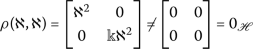Therefore, $$(\Upsilon,\mathscr{H},\rho )$$ is not a $$\mathscr{C}^{*}$$-algebra-valued b-metric space.

### Definition 2.4

A sequence $$\{\aleph _{\alpha}\}$$ in $$(\Upsilon, \mathscr{H},\rho )$$ is called convergent (with respect to $$\mathscr{H}$$) to a point $$\aleph \in \Upsilon$$ if, for given $$\epsilon >0$$, there exists $$\mathfrak{k}\in \mathbb{N}$$ such that $$\Vert \rho (\aleph _{\alpha},\aleph )-\rho (\aleph,\aleph \Vert <\epsilon$$, for all $$\alpha >\mathfrak{k}$$. We denote it by

\begin{aligned} \lim_{\alpha \to \infty}\rho (\aleph _{\alpha},\aleph )=\rho ( \aleph, \aleph ). \end{aligned}

### Definition 2.5

A sequence $$\{\aleph _{\alpha}\}$$ in $$(\Upsilon,\mathscr{H},\rho )$$ is called Cauchy (with respect to $$\mathscr{H}$$) if $$\lim\limits_{\alpha \to \infty}\rho (\aleph _{\alpha},\aleph _{\mathfrak{m}})$$ exists and it is finite.

### Definition 2.6

The triplet $$(\Upsilon,\mathscr{H},\rho )$$ is called a complete $$C^{*}$$-algebra-valued partial b-metric space if every Cauchy sequence in ϒ is convergent to some point in ϒ such that

\begin{aligned} \lim_{\alpha \to \infty}\rho (\aleph _{\alpha},\aleph _{ \mathfrak{m}})= \lim_{\alpha \to \infty}\rho (\aleph _{\alpha}, \aleph )=\rho (\aleph, \aleph ). \end{aligned}

### Definition 2.7

()

Let ϒ be a nonempty set. An element $$(\aleph,\varpi ) \in \Upsilon \times \Upsilon$$ is said to be

(1) a coupled fixed point of the mapping $$\mathcal{T}:\Upsilon \times \Upsilon \to \Upsilon$$ if $$\mathcal{T}(\aleph,\varpi ) = \aleph$$ and $$\mathcal{T}(\varpi,\aleph ) = \varpi$$.

(2) a coupled coincidence point of the mapping $$\mathcal{T}:\Upsilon \times \Upsilon \to \Upsilon$$ and $$g: \Upsilon \to \Upsilon$$ if $$\mathcal{T}(\aleph,\varpi ) = g\aleph$$ and $$\mathcal{T}(\varpi,\aleph ) = g\varpi$$. In this case $$(g\aleph,g\varpi )$$ is said to be a coupled point of coincidence.

(3) a common coupled fixed point of the mapping $$\mathcal{T}:\Upsilon \times \Upsilon \to \Upsilon$$ and $$g: \Upsilon \to \Upsilon$$ if $$\mathcal{T}(\aleph,\varpi ) = g\aleph = \aleph$$ and $$\mathcal{T}(\varpi,\aleph ) = g\varpi = \varpi$$.

Note that Definition 2.7(3) reduces to Definition 2.7(1) if the mapping g is the identity mapping.

### Definition 2.8

()

The mappings $$\mathcal{T}:\Upsilon \times \Upsilon \to \Upsilon$$ and $$g: \Upsilon \to \Upsilon$$ is said to be ω-compatible if $$g(\mathcal{T}(\aleph,\varpi )) = \mathcal{T}(g\aleph,g\varpi )$$ whenever $$g\aleph = \mathcal{T}(\aleph,\varpi )$$ and $$g\varpi = \mathcal{T}(\varpi,\aleph )$$.

In this paper, we prove coupled fixed point theorems on $$C^{*}$$-algebra-valued partial b-metric space.

## Main results

In this section we shall prove some common coupled fixed point theorems for different contractive mappings in the setting of $$\mathscr{C}^{\star}$$-algebra-valued partial b-metric spaces. Now we give our main results.

### Theorem 3.1

Let $$(\Upsilon,\mathscr{H}, \rho )$$ be a complete $$\mathscr{C}^{\star}$$-algebra-valued partial b-metric space with coefficient s. Suppose that the mappings $$\mathcal{T}:\Upsilon \times \Upsilon \to \Upsilon$$ and $$g: \Upsilon \to \Upsilon$$ satisfy the following condition:

\begin{aligned} \rho \bigl(\mathcal{T}(\aleph,\varpi ),\mathcal{T}(\imath,v)\bigr) \preceq r^{ \star}\rho (g\aleph,g\imath )r + r^{\star}\rho (g\varpi,gv)r\quad \textit{for any } \aleph,\varpi,\imath,v \in \Upsilon, \end{aligned}
(1)

where $$r \in \mathscr{H}$$ with $$\Vert r \Vert < \frac{1}{\surd{2}}$$ and $$\Vert s\Vert \Vert \sqrt{2}r\Vert ^{2}<1$$. If $$\mathcal{T} (\Upsilon \times \Upsilon ) \subseteq g(\Upsilon )$$ and $$g(\Upsilon )$$ is complete in ϒ, then $$\mathcal{T}$$ and g have a coupled coincidence point and $$\rho (g\aleph, g\aleph )=0_{\mathscr{H}}$$ and $$\rho (g\varpi, g\varpi )=0_{\mathscr{H}}$$. Moreover, if $$\mathcal{T}$$ and g are ω-compatible, then they have a unique common coupled fixed point in ϒ.

### Proof

Take $$\aleph _{0},\varpi _{0} \in \Upsilon$$, and let $$g(\aleph _{1}) = \mathcal{T}(\aleph _{0},\varpi _{0})$$ and $$g(\varpi _{1}) = \mathcal{T}(\varpi _{0},\aleph _{0})$$. One can obtain two sequences $$\{\aleph _{\alpha}\}$$ and $$\{\varpi _{\alpha}\}$$ by continuing this process such that $$g(\aleph _{\alpha +1}) = \mathcal{T}(\aleph _{\alpha},\varpi _{ \alpha})$$ and $$g(\varpi _{\alpha +1}) = \mathcal{T}(\varpi _{\alpha},\aleph _{ \alpha})$$. From (1), we get

\begin{aligned} \rho (g\aleph _{\alpha}, g\aleph _{\alpha +1}) &= \rho \bigl( \mathcal{T}( \aleph _{\alpha -1},\varpi _{\alpha -1}),\mathcal{T}(\aleph _{\alpha}, \varpi _{\alpha})\bigr) \\ &\preceq r^{\star}\bigl(\rho (g\aleph _{\alpha -1},g\aleph _{\alpha})\bigr)r +r^{ \star}\bigl(\rho (g\varpi _{\alpha -1},g \varpi _{\alpha})\bigr)r \\ &\preceq r^{\star}\bigl(\rho (g\aleph _{\alpha -1},g\aleph _{\alpha})\bigr) +\bigl( \rho (g\varpi _{\alpha -1},g\varpi _{\alpha})\bigr)r . \end{aligned}
(2)

Similarly,

\begin{aligned} \rho (g\varpi _{\alpha}, g\varpi _{\alpha +1}) &= \rho \bigl( \mathcal{T}( \varpi _{\alpha -1},\aleph _{\alpha -1}),\mathcal{T}(\varpi _{\alpha}, \aleph _{\alpha})\bigr) \\ &\preceq r^{\star}\bigl(\rho (g\varpi _{\alpha -1},g\varpi _{\alpha})\bigr)r +r^{ \star}\bigl(\rho (g\aleph _{\alpha -1},g \aleph _{\alpha})\bigr)r \\ &\preceq r^{\star}\bigl(\rho (g\varpi _{\alpha -1},g\varpi _{\alpha})\bigr) +\bigl( \rho (g\aleph _{\alpha -1},g\aleph _{\alpha})\bigr)r . \end{aligned}
(3)

Let

\begin{aligned} \Im _{\alpha} = \rho (g\aleph _{\alpha},g\aleph _{\alpha +1}) + \rho (g \varpi _{\alpha},g\varpi _{\alpha +1}), \end{aligned}

and now from (2) and (3), we have

\begin{aligned} \Im _{\alpha} &= \rho (g\aleph _{\alpha},g\aleph _{\alpha +1}) + \rho (g\varpi _{\alpha},g\varpi _{\alpha +1}) \\ &\preceq r^{\star}\bigl(\rho (g\aleph _{\alpha -1},g\aleph _{\alpha}) + \rho (g\varpi _{\alpha -1},g\varpi _{\alpha})\bigr)r + r^{\star}\bigl(\rho (g \varpi _{\alpha -1},g\varpi _{\alpha}) +\rho (g\aleph _{\alpha -1},g \aleph _{\alpha})\bigr)r \\ &\preceq (\sqrt{2}r)^{\star}\bigl(\rho (g\aleph _{\alpha -1},g\aleph _{ \alpha}) +\rho (g\varpi _{\alpha -1},g\varpi _{\alpha})\bigr) ( \sqrt{2}r) \\ & \preceq (\sqrt{2}r)^{\star} \Im _{\alpha -1}(\sqrt{2}r), \end{aligned}

which, together with the property: if $$s, \mathfrak{t} \in \mathscr{H}_{\eth}$$, then $$s \preceq \mathfrak{t}$$ implies $$r^{\star}sr \preceq r^{\star}\mathfrak{t}r$$ (Theorem 2.2.5 in ), yields that for each $$\alpha \in \mathbb{N}$$,

\begin{aligned} 0_{\mathscr{H}} \preceq \Im _{\alpha} \preceq (\sqrt{2}r)^{\star}\Im _{ \alpha -1}(\sqrt{2}r) \preceq \dots \preceq \bigl[(\sqrt{2}r)^{\star} \bigr]^{ \alpha}\Im _{0}(\sqrt{2}r)^{\alpha}. \end{aligned}

If $$\Im _{0} = 0_{\mathscr{H}}$$, then we know that $$\mathcal{T}$$ and g have a coupled coincidence point $$(\aleph _{0}, \varpi _{0})$$. Now, letting $$0_{\mathscr{H}} \prec \Im _{0}$$, we can obtain for $$\wp >\alpha$$, $$\alpha, \wp \in \mathbb{N}$$,

\begin{aligned} \rho (g\aleph _{\alpha},g\aleph _{\wp}) \preceq{}& s\bigl(\rho (g \aleph _{ \alpha},g\aleph _{\alpha +1}) + \rho (g\aleph _{\alpha +1},g \aleph _{ \wp})\bigr)-\rho (g\aleph _{\alpha +1},g\aleph _{\alpha +1}) \\ \preceq{}& s\rho (g\aleph _{\alpha},g\aleph _{\alpha +1})+ s^{2} \bigl(\rho (g \aleph _{\alpha +1},g\aleph _{\alpha +2})+\rho (g\aleph _{\alpha +2},g \aleph _{\wp})\bigr) \\ &{} -\rho (g\aleph _{\alpha +1},g\aleph _{\alpha +1})-\rho (g \aleph _{\alpha +2},g\aleph _{\alpha +2}) \\ \preceq{}& s\rho (g\aleph _{\alpha},g\aleph _{\alpha +1})+ s^{2} \rho (g \aleph _{\alpha +1},g\aleph _{\alpha +2}) \\ &{} +\cdots +s^{\wp -\alpha -1}\bigl(\rho (g\aleph _{\wp -2},g\aleph _{ \wp -1})+\rho (g\aleph _{\wp -1},g\aleph _{\wp})\bigr) \\ &{} -\rho (g\aleph _{\alpha +1},g\aleph _{\alpha +1})-\cdots - \rho (g\aleph _{\wp -1},g\aleph _{\wp -1}) \\ \preceq{}& s\rho (g\aleph _{\alpha},g\aleph _{\alpha +1})+ s^{2} \rho (g \aleph _{\alpha +1},g\aleph _{\alpha +2}) \\ &{} +\cdots +s^{\wp -\alpha -1}\rho (g\aleph _{\wp -2},g\aleph _{ \wp -1})+s^{\wp -\alpha -1}\rho (g\aleph _{\wp -1},g\aleph _{\wp}), \\ \rho (g\varpi _{\alpha},g\varpi _{\wp}) \preceq{}& s\bigl(\rho (g \varpi _{ \alpha},g\varpi _{\alpha +1}) + \rho (g\varpi _{\alpha +1},g \varpi _{ \wp})\bigr)-\rho (g\varpi _{\alpha +1},g\varpi _{\alpha +1}) \\ \preceq{}& s\rho (g\varpi _{\alpha},g\varpi _{\alpha +1})+ s^{2} \bigl(\rho (g \varpi _{\alpha +1},g\varpi _{\alpha +2})+\rho (g\varpi _{\alpha +2},g \varpi _{\wp})\bigr) \\ &{} -\rho (g\varpi _{\alpha +1},g\varpi _{\alpha +1})-\rho (g \varpi _{\alpha +2},g\varpi _{\alpha +2}) \\ &\preceq s\rho (g\varpi _{\alpha},g\varpi _{\alpha +1})+ s^{2} \rho (g \varpi _{\alpha +1},g\varpi _{\alpha +2}) \\ &{} +\cdots +s^{\wp -\alpha -1}\bigl(\rho (g\varpi _{\wp -2},g\varpi _{ \wp -1})+\rho (g\varpi _{\wp -1},g\varpi _{\wp})\bigr) \\ &{} -\rho (g\varpi _{\alpha +1},g\varpi _{\alpha +1})-\cdots - \rho (g\varpi _{\wp -1},g\varpi _{\wp -1}) \\ &\preceq s\rho (g\varpi _{\alpha},g\varpi _{\alpha +1})+ s^{2} \rho (g \varpi _{\alpha +1},g\varpi _{\alpha +2}) \\ &{} +\cdots +s^{\wp -\alpha -1}\rho (g\varpi _{\wp -2},g\varpi _{ \wp -1})+s^{\wp -\alpha -1}\rho (g\varpi _{\wp -1},g\varpi _{\wp}). \end{aligned}

Consequently,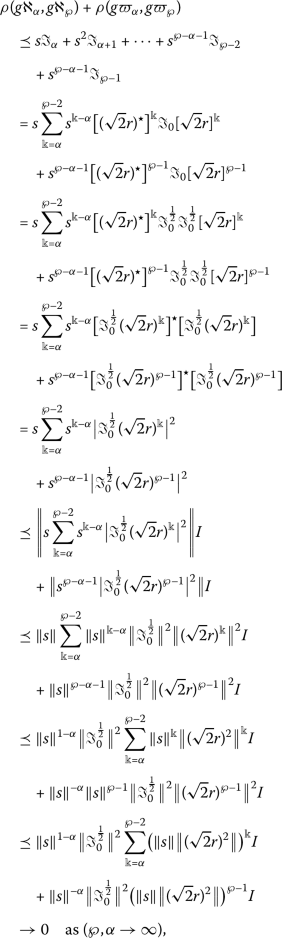(4)

which follows from the observation that the sum in the first term is a geometric series and $$\Vert s\Vert \Vert (\sqrt{2}r)^{2}\Vert <1$$ implies that $$(\Vert s\Vert \Vert (\sqrt{2}r)^{2}\Vert )^{\wp -1}\to 0$$ and $$(\Vert s\Vert \Vert (\sqrt{2}r)^{2}\Vert )^{\alpha}\to 0$$. This proves that $$\{g\aleph _{\alpha}\}$$ and $$\{g\varpi _{\alpha}\}$$ are Cauchy sequences in $$g(\Upsilon )$$. Since $$\{g\varpi _{\alpha}\}$$ is complete, there exist $$\aleph,\varpi \in \Upsilon$$ such that

\begin{aligned} &\rho (g\aleph, g\aleph )=\lim_{n \to \infty}\rho (g\aleph _{\alpha},g \aleph )=\lim_{n \to \infty}\rho (g\aleph _{\alpha},g\aleph _{m}), \\ &\rho (g\varpi, g\varpi )=\lim_{n \to \infty}\rho (g\varpi _{\alpha},g \varpi )=\lim_{n \to \infty}\rho (g\varpi _{\alpha},g\varpi _{m}). \end{aligned}

By (4), we have

\begin{aligned} \lim_{n \to \infty}\rho (g\aleph _{\alpha},g\aleph )+\lim _{n \to \infty}\rho (g\varpi _{\alpha},g\varpi )=\rho (g\aleph, g \aleph )+ \rho (g\varpi, g\varpi )=0_{\mathscr{H}}. \end{aligned}

Now, we prove that $$\mathcal{T}(\aleph,\varpi ) = g\aleph$$ and $$\mathcal{T}(\varpi,\aleph ) =g\varpi$$. For that we have

\begin{aligned} \rho \bigl(\mathcal{T}(\aleph,\varpi ), g\aleph \bigr)&\preceq s\bigl(\rho \bigl( \mathcal{T}(\aleph,\varpi ), g\aleph _{\alpha +1}\bigr) + \rho ( g \aleph _{\alpha +1}, g\aleph )\bigr)-\rho (g\aleph _{\alpha +1}, g\aleph _{ \alpha +1}) \\ &\preceq s\rho \bigl(\mathcal{T}(\aleph,\varpi ), \mathcal{T}(\aleph _{ \alpha},\varpi _{\alpha})\bigr)+s\rho ( g\aleph _{\alpha +1}, g \aleph ) \\ &\preceq sr^{\star} \rho (g\aleph _{\alpha}, g\aleph )r+sr^{\star} \rho (g\varpi _{\alpha}, g\varpi )r+s\rho ( g\aleph _{\alpha +1}, g \aleph ). \end{aligned}

Taking the limit as $$\alpha \rightarrow \infty$$ in the above relation, we get $$\rho (\mathcal{T}(\aleph,\varpi ), g\aleph ) = 0_{\mathscr{H}}$$ and hence $$\mathcal{T}(\aleph,\varpi ) = g\aleph$$. Similarly, $$\mathcal{T}(\varpi,\aleph ) = g\varpi$$. Therefore, $$\mathcal{T}$$ and g have a coupled coincidence point $$(\aleph,\varpi )$$.

Now if $$\mathcal{T}$$ and g have a coupled coincidence point $$(\aleph ',\varpi ')$$, then

\begin{aligned} &\rho \bigl(g\aleph,g\aleph '\bigr) = \rho \bigl(\mathcal{T}(\aleph, \varpi ), \mathcal{T}\bigl(\aleph ',\varpi '\bigr)\bigr) \preceq r^{\star}\rho \bigl(g\aleph,g \aleph '\bigr)r + r^{\star}\rho \bigl(g\varpi,g\varpi '\bigr)r, \\ &\rho \bigl(g\varpi,g\varpi '\bigr) = \rho \bigl(\mathcal{T}(\varpi, \aleph ), \mathcal{T}\bigl(\varpi ',\aleph '\bigr)\bigr) \preceq r^{\star}\rho \bigl(g\varpi,g \varpi '\bigr)r + r^{\star}\rho \bigl(g\aleph,g\aleph '\bigr)r, \end{aligned}

and hence

\begin{aligned} \rho \bigl(g\aleph,g\aleph '\bigr) + \rho \bigl(g\varpi,g\varpi '\bigr) \preceq (\sqrt{2}r)^{ \star}\bigl(\rho \bigl(g\aleph,g \aleph '\bigr) + \rho \bigl(g\varpi,g\varpi '\bigr) \bigr) (\sqrt{2}r), \end{aligned}

which further induces that

\begin{aligned} \bigl\Vert \rho \bigl(g\aleph,g\aleph '\bigr) + \rho \bigl(g \varpi,g\varpi '\bigr) \bigr\Vert \preceq \bigl\Vert (\sqrt{2}r) \bigr\Vert ^{2} \bigl\Vert \rho \bigl(g\aleph,g\aleph ' \bigr) + \rho \bigl(g\varpi,g\varpi '\bigr) \bigr\Vert . \end{aligned}

Since $$\Vert (\sqrt{2}r)\Vert \lessdot 1$$, then $$\Vert \rho (g\aleph,g\aleph ') + \rho (g\varpi,g\varpi ')\Vert = 0$$. Hence, we get $$g\aleph = g\aleph '$$ and $$g\varpi = g\varpi '$$. Similarly, we can prove that $$g\aleph = g\varpi '$$ and $$g\varpi = g\aleph '$$. Then $$\mathcal{T}$$ and g have a unique coupled point of coincidence $$(g\aleph,g\aleph )$$. Moreover, if $$v = g\aleph$$, then $$v= g\aleph = \mathcal{T}(\aleph, \aleph )$$. Since $$\mathcal{T}$$ and g are ω-compatible,

\begin{aligned} gv= g(g\aleph ) = g\bigl(\mathcal{T}(\aleph, \aleph )\bigr) = \mathcal{T}(g \aleph, g\aleph )= \mathcal{T}(v, v), \end{aligned}

which means that $$\mathcal{T}$$ and g have a coupled point of coincidence $$(gv, gv)$$. By the uniqueness, we know $$gv=g\aleph$$, which yields that $$v=gv=\mathcal{T}(v, v)$$. Therefore, F and g have a unique common coupled fixed point $$(v, v)$$. □

### Example 3.2

Let $$\Upsilon = \mathcal{R}$$ and $$\mathscr{H} = \mathcal{M}_{2}(\mathbb{C})$$ and the map $$\rho: \Upsilon \times \Upsilon \rightarrowtail \mathscr{H}$$ is defined bywhere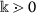is a constant. Then $$(\Upsilon,\mathscr{H}, \rho )$$ is a complete $$\mathscr{C}^{\star}$$-algebra-valued partial b-metric space. Consider the mappings $$\mathcal{T}: \Upsilon \times \Upsilon \to \Upsilon$$ with $$\mathcal{T}(\aleph,\varpi ) = \frac{\aleph + \varpi}{2}$$ and $$g:\Upsilon \to \Upsilon$$ with $$g(\aleph )=2\aleph$$. Set $$\lambda \in \mathbb{C}$$ with $$\vert \lambda \vert <\frac{1}{\surd 2}$$, and $r=\left[\begin{array}{cc}\lambda & 0\\ 0& \lambda \end{array}\right]$, then $$r \in \mathscr{H}$$ and $$\Vert r \Vert _{\infty} = \vert \lambda \vert$$. Clearly, $$\mathcal{T}$$ and g are ω-compatible. Moreover, one can verify that $$\mathcal{T}$$ satisfies the contractivity condition

\begin{aligned} \rho \bigl(\mathcal{T}(\aleph,\varpi ),\mathcal{T}(u,v)\bigr) \preceq r^{\star} \mathcal{T}(\aleph,u)r + r^{\star}\mathcal{T}(\varpi,v)r \quad\text{for any } \aleph,\varpi,u,v \in \Upsilon. \end{aligned}

In this case, $$(0, 0)$$ is a coupled coincidence point of $$\mathcal{T}$$ and g. Moreover, $$(0, 0)$$ is a unique common coupled fixed point of $$\mathcal{T}$$ and g.

### Corollary 3.3

Let $$(\Upsilon,\mathscr{H}, \rho )$$ be a complete $$\mathscr{C}^{\star}$$-algebra-valued partial b-metric space with coefficient s. Suppose that the mapping $$\mathcal{T}: \Upsilon \times \Upsilon \to \Upsilon$$ satisfies the following condition:

\begin{aligned} \rho \bigl(\mathcal{T}(\aleph,\varpi ),\mathcal{T}(u,v)\bigr) \preceq r^{\star} \rho (\aleph,u)r + r^{\star}\rho (\varpi,v)r \quad\textit{for any } \aleph,\varpi,u,v \in \Upsilon, \end{aligned}
(5)

where $$r \in \mathscr{H}$$ with $$\Vert r \Vert <\frac{1}{\surd 2}$$ and $$\Vert s\Vert \Vert \sqrt{2}r\Vert ^{2}<1$$. Then $$\mathcal{T}$$ has a unique coupled fixed point.

Before going to another theorem, we recall the following lemma of .

### Lemma 3.4

Suppose that $$\mathscr{H}$$ is a unital $$\mathscr{C}^{\star}$$-algebra with a unit $$1_{\mathscr{H}}$$.

1. 1.

If $$r \in \mathscr{H}_{+}$$ with $$\Vert r \Vert < \frac{1}{2}$$, then $$1_{\mathscr{H}} - r$$ is invertible.

2. 2.

If $$r,\mathfrak{b} \in \mathscr{H}_{+}$$ and $$r\mathfrak{b} = \mathfrak{b}r$$, then $$0_{\mathscr{H}} \preceq r\mathfrak{b}$$.

3. 3.

If $$r,\mathfrak{b} \in \mathscr{H}_{\eth}$$ and $$\mathfrak{t} \in \mathscr{H}_{+}^{\prime }$$ then $$r \preceq \mathfrak{b}$$ deduces $$\mathfrak{t}r \preceq \mathfrak{t}\mathfrak{b}$$, where $$\mathscr{H}_{+}^{\prime } = \mathscr{H}_{+} \cap \mathscr{H}^{\prime }$$.

### Theorem 3.5

Let $$(\Upsilon,\mathscr{H}, \rho )$$ be a complete $$\mathscr{C}^{\star}$$-algebra-valued partial b-metric space with coefficient s. Suppose that the mappings $$\mathcal{T}: \Upsilon \times \Upsilon \to \Upsilon$$ and $$g: \Upsilon \to \Upsilon$$ satisfies the following condition:

\begin{aligned} \rho \bigl(\mathcal{T}(\aleph,\varpi ),\mathcal{T}(u,v)\bigr) \preceq r\rho \bigl( \mathcal{T}(\aleph,\varpi ),g\aleph \bigr) + \mathfrak{b}\rho \bigl( \mathcal{T}(u,v),gu\bigr), \end{aligned}
(6)

for any $$\aleph,\varpi,u,v \in \Upsilon$$, where $$r,\mathfrak{b}\in \mathscr{H}_{+}^{\prime }$$ with $$\Vert r \Vert + \Vert \mathfrak{b} \Vert <1$$. If $$\mathcal{T}(\Upsilon \times \Upsilon ) \subseteq g(\Upsilon )$$ and $$g(\Upsilon )$$ is complete in ϒ, then $$\mathcal{T}$$ and g have a coupled coincidence point and $$\rho (g\aleph, g\aleph )=0_{\mathscr{H}}$$ and $$\rho (g\varpi, g\varpi )=0_{\mathscr{H}}$$. Moreover, if $$\mathcal{T}$$ and g are ω-compatible, then they have unique common coupled fixed point in ϒ.

### Proof

As in the proof of Theorem 3.1, construct two sequences $$\{\aleph _{\alpha}\}$$ and $$\{\varpi _{\alpha}\}$$ in ϒ such that $$g\aleph _{\alpha +1} = \mathcal{T}(\aleph _{\alpha}, \varpi _{\alpha})$$ and $$g\varpi _{\alpha +1} = \mathcal{T}(\varpi _{\alpha}, \aleph _{\alpha})$$. Then by applying (6), we have

\begin{aligned} &(1_{\mathscr{H}} - \mathfrak{b})\rho (g\aleph _{\alpha},g\aleph _{ \alpha +1}) \preceq r\rho (g\aleph _{\alpha},g\aleph _{\alpha -1}), \\ &(1_{\mathscr{H}} - \mathfrak{b})\rho (g\varpi _{\alpha},g\varpi _{ \alpha +1}) \preceq r\rho (g\varpi _{\alpha},g\varpi _{\alpha -1}). \end{aligned}

Since $$r,\mathfrak{b} \in \mathscr{H}_{+}^{\prime }$$ with $$\Vert r \Vert + \Vert \mathfrak{b} \Vert < 1$$, we have $$1_{\mathscr{H}} - \mathfrak{b}$$ is invertible and $$(1_{\mathscr{H}} - \mathfrak{b})^{-1}r \in \mathscr{H}_{+}^{\prime }$$. Therefore

\begin{aligned} &\rho (g\aleph _{\alpha},g\aleph _{\alpha +1}) \preceq (1_{ \mathscr{H}} - \mathfrak{b})^{-1} r\rho (g\aleph _{\alpha},g\aleph _{ \alpha -1}), \\ &\rho (g\varpi _{\alpha},g\varpi _{\alpha +1}) \preceq (1_{ \mathscr{H}} - \mathfrak{b})^{-1} r\rho (g\varpi _{\alpha},g\varpi _{ \alpha -1}). \end{aligned}

Then

\begin{aligned} &\bigl\Vert \rho (g\aleph _{\alpha},g\aleph _{\alpha +1}) \bigr\Vert \preceq \bigl\Vert (1_{\mathscr{H}} - \mathfrak{b})^{-1} r \bigr\Vert \bigl\Vert \rho (g \aleph _{\alpha},g\aleph _{\alpha -1}) \bigr\Vert , \\ &\bigl\Vert \rho (g\varpi _{\alpha},g\varpi _{\alpha +1}) \bigr\Vert \preceq \bigl\Vert (1_{\mathscr{H}} - \mathfrak{b})^{-1} r \bigr\Vert \bigl\Vert \rho (g \varpi _{\alpha},g\varpi _{\alpha -1}) \bigr\Vert . \end{aligned}

It follows from the fact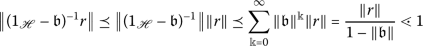that $$\{g\aleph _{\alpha}\}$$ and $$\{g\varpi _{\alpha}\}$$ are Cauchy sequences in $$g(\Upsilon )$$ and therefore, by the completeness of $$g(\Upsilon )$$, there are $$\aleph,\varpi \in \Upsilon$$ such that $$\lim\limits_{\alpha \rightarrowtail \infty}g\aleph _{\alpha}=g\aleph$$ and

\begin{aligned} \rho (g\aleph, g\aleph )=\lim_{n \to \infty}\rho (g\aleph _{\alpha},g \aleph )=\lim_{n \to \infty}\rho (g\aleph _{\alpha},g\aleph _{\alpha})=0_{ \mathscr{H}}, \end{aligned}

$$\lim_{\alpha \rightarrowtail \infty}g\varpi _{\alpha} =g\varpi$$ and

\begin{aligned} \rho (g\varpi, g\varpi )=\lim_{n \to \infty}\rho (g\varpi _{\alpha},g \varpi )=\lim_{n \to \infty}\rho (g\varpi _{\alpha},g\varpi _{\alpha})=0_{ \mathscr{H}}. \end{aligned}

Since

\begin{aligned} \rho \bigl(\mathcal{T}(\aleph,\varpi ),g\aleph \bigr) &\preceq s\bigl[\rho \bigl(g \aleph _{\alpha +1},\mathcal{T}(\aleph,\varpi )\bigr) + \rho (g\aleph _{ \alpha +1},g\aleph )\bigr]-\rho (g\aleph _{\alpha +1},g\aleph _{\alpha +1}) \\ &\preceq s\bigl[\rho \bigl(g\aleph _{\alpha +1},\mathcal{T}(\aleph,\varpi ) \bigr) + \rho (g\aleph _{\alpha +1},g\aleph )\bigr] \\ &=s\bigl(\rho \bigl(\mathcal{T}(\aleph _{\alpha},\varpi _{\alpha}), \mathcal{T}( \aleph,\varpi )\bigr) + \rho (g\aleph _{\alpha +1},g\aleph )\bigr) \\ &\preceq sr\rho \bigl(\mathcal{T}(\aleph _{\alpha},\varpi _{\alpha}),g \aleph _{\alpha}\bigr)+s\mathfrak{b}\rho \bigl(\mathcal{T}(\aleph,\varpi ),g \aleph \bigr) + s\rho (g\aleph _{\alpha +1},g\aleph ) \\ &\preceq sr \rho (g\aleph _{\alpha +1},g\aleph _{\alpha}) + s \mathfrak{b}\rho \bigl(\mathcal{T}(\aleph,\varpi ),g\aleph \bigr) + s\rho (g \aleph _{\alpha +1},g\aleph ), \end{aligned}

hence

\begin{aligned} \rho \bigl(\mathcal{T}(\aleph,\varpi ),g\aleph \bigr) \preceq (1 - s \mathfrak{b})^{-1} sr \rho (g\aleph _{\alpha +1},g\aleph _{\alpha}) + (1 - s\mathfrak{b})^{-1}s\rho (g\aleph _{\alpha +1},g\aleph _{\alpha}). \end{aligned}

Then $$\rho (\mathcal{T}(\aleph,\varpi ),g\aleph )=0_{\mathscr{H}}$$, or equivalently, $$\mathcal{T}(\aleph,\varpi )=g\aleph$$. Similarly, one can obtain $$\mathcal{T}(\varpi,\aleph ) = g\varpi$$.

Now, if $$(\aleph ',\varpi ')$$ is another coupled coincidence point of $$\mathcal{T}$$ and g, then according to (6), we obtain

\begin{aligned} \rho \bigl(g\aleph ',g\aleph \bigr) &\preceq \rho \bigl(\mathcal{T} \bigl(\aleph ',\varpi '\bigr), \mathcal{T}(\aleph,\varpi )\bigr) \\ &\preceq r\rho \bigl(\mathcal{T}\bigl(\aleph ',\varpi '\bigr),g\aleph '\bigr) + \mathfrak{b}\rho \bigl( \mathcal{T}(\aleph,\varpi ),g\aleph \bigr) \\ &=r\rho \bigl(g\aleph ',g\aleph '\bigr)+\mathfrak{b} \rho (g\aleph,g\aleph )= 0_{ \mathscr{H}} \end{aligned}

and

\begin{aligned} \rho \bigl(g\varpi ',g\varpi \bigr) &\preceq \rho \bigl(\mathcal{T} \bigl(\varpi ',\aleph '\bigr), \mathcal{T}(\varpi,\aleph )\bigr) \\ &\preceq r\rho \bigl(\mathcal{T}\bigl(\varpi ',\aleph '\bigr),g\varpi '\bigr)+ \mathfrak{b}\rho \bigl( \mathcal{T}(\varpi,\aleph ),g\varpi \bigr) \\ &=r\rho \bigl(g\varpi ',g\varpi '\bigr)+\mathfrak{b} \rho (g\varpi,g\varpi ) = 0_{ \mathscr{H}}, \end{aligned}

which implies that $$g\aleph '=g\aleph$$ and $$g\varpi '=g\varpi$$. Similarly, we have $$g\aleph '=g\varpi$$ and $$g\varpi '=g\aleph$$. Hence $$\mathcal{T}$$ and g have a unique coupled point of coincidence $$(g\aleph, g\aleph )$$. Moreover, we can show that $$\mathcal{T}$$ and g have a unique common coupled fixed point. □

### Theorem 3.6

Let $$(\Upsilon,\mathscr{H}, \rho )$$ be a complete $$\mathscr{C}^{\star}$$-algebra-valued partial b-metric space with coefficient s. Suppose that the mappings $$\mathcal{T}: \Upsilon \times \Upsilon \to \Upsilon$$ and $$g: \Upsilon \to \Upsilon$$ satisfies the following condition:

\begin{aligned} \rho \bigl(\mathcal{T}(\aleph,\varpi ),\mathcal{T}(u,v)\bigr) \preceq r\rho \bigl( \mathcal{T}(\aleph,\varpi ),gu\bigr) + \mathfrak{b}\rho \bigl( \mathcal{T}(u,v),g \aleph \bigr) \end{aligned}
(7)

for any $$\aleph,\varpi,u,v \in \Upsilon$$, where $$r,\mathfrak{b}\in \mathscr{H}_{+}'$$ with $$\Vert r \Vert + \Vert \mathfrak{b} \Vert <1$$ and $$\Vert sr\Vert +\Vert s\mathfrak{b}\Vert <1$$. If $$\mathcal{T}(\Upsilon \times \Upsilon ) \subseteq g(\Upsilon )$$ and $$g(\Upsilon )$$ is complete in ϒ, then $$\mathcal{T}$$ and g have a coupled coincidence point and $$\rho (g\aleph, g\aleph )=0_{\mathscr{H}}$$ and $$\rho (g\varpi, g\varpi )=0_{\mathscr{H}}$$. Moreover, if $$\mathcal{T}$$ and g are ω-compatible, then they have unique common coupled fixed point in ϒ.

### Proof

Following a similar argument given in the proof of Theorem 3.1, we construct two sequences $$\{\aleph _{\alpha}\}$$ and $$\{\varpi _{\alpha}\}$$ in ϒ such that $$g(\aleph _{\alpha +1}) = \mathcal{T}(\aleph _{\alpha},\varpi _{ \alpha})$$ and $$g(\varpi _{\alpha +1}) = \mathcal{T}(\varpi _{\alpha},\aleph _{ \alpha})$$. Now, from (7), we have

\begin{aligned} \rho (g\aleph _{\alpha}, g\aleph _{\alpha +1}) ={}& \rho \bigl( \mathcal{T}( \aleph _{\alpha -1},\varpi _{\alpha -1}),\mathcal{T}(\aleph _{\alpha}, \varpi _{\alpha})\bigr) \\ \preceq{}& r\rho \bigl(\mathcal{T}(\aleph _{\alpha -1},\varpi _{\alpha -1}),g \aleph _{\alpha}\bigr) + \mathfrak{b}\rho \bigl( \mathcal{T}(\aleph _{\alpha}, \varpi _{\alpha}),g\aleph _{\alpha -1} \bigr) \\ \preceq{}& r\rho (g\aleph _{\alpha},g\aleph _{\alpha})+ \mathfrak{b} \rho (g\aleph _{\alpha +1},g\aleph _{\alpha -1}) \\ \preceq{}& r\rho (g\aleph _{\alpha +1},g\aleph _{\alpha})+ s \mathfrak{b} \rho (g\aleph _{\alpha +1},g\aleph _{\alpha}) + s \mathfrak{b}\rho (g \aleph _{\alpha},g\aleph _{\alpha -1}) \\ &{}-\mathfrak{b}\rho (\rho (g\aleph _{\alpha},g\aleph _{\alpha}) \\ \preceq{}& r\rho (g\aleph _{\alpha +1},g\aleph _{\alpha})+ s \mathfrak{b} \rho (g\aleph _{\alpha +1},g\aleph _{\alpha}) + s \mathfrak{b}\rho (g \aleph _{\alpha},g\aleph _{\alpha -1}), \end{aligned}

from which it follows that

\begin{aligned} \bigl(1_{\mathscr{H}}-(r+s\mathfrak{b})\bigr)\rho (g\aleph _{\alpha}, g \aleph _{ \alpha +1})\preceq s\mathfrak{b}\rho (g\aleph _{\alpha},g\aleph _{ \alpha -1}). \end{aligned}
(8)

Because of the symmetry in (7), we have

\begin{aligned} \rho (g\aleph _{\alpha +1}, g\aleph _{\alpha}) ={}& \rho \bigl( \mathcal{T}( \aleph _{\alpha},\varpi _{\alpha}),\mathcal{T}(\aleph _{\alpha -1}, \varpi _{\alpha -1})\bigr) \\ \preceq{}& r\rho \bigl(\mathcal{T}(\aleph _{\alpha},\varpi _{\alpha}),g \aleph _{\alpha -1}\bigr) + \mathfrak{b}\rho \bigl(\mathcal{T}(\aleph _{\alpha -1}, \varpi _{\alpha -1}),g\aleph _{\alpha}\bigr) \\ \preceq{}& r\rho (g\aleph _{\alpha +1},g\aleph _{\alpha -1})+ \mathfrak{b} \rho (g\aleph _{\alpha},g\aleph _{\alpha}) \\ \preceq{}& sr\rho (g\aleph _{\alpha +1},g\aleph _{\alpha}) + sr\rho (g \aleph _{\alpha},g\aleph _{\alpha -1})-r\rho (g\aleph _{\alpha},g \aleph _{\alpha}) \\ &{} +\mathfrak{b}\rho (g\aleph _{\alpha +1},g\aleph _{\alpha}) \\ \preceq{}& sr\rho (g\aleph _{\alpha +1},g\aleph _{\alpha}) + sr\rho (g \aleph _{\alpha},g\aleph _{\alpha -1})+ \mathfrak{b}\rho (g\aleph _{ \alpha +1},g\aleph _{\alpha}), \end{aligned}

that is,

\begin{aligned} \bigl(1_{\mathscr{H}}-(sr+\mathfrak{b})\bigr)\rho (g\aleph _{\alpha}, g \aleph _{ \alpha +1})\preceq sr\rho (g\aleph _{\alpha},g\aleph _{\alpha -1}). \end{aligned}
(9)

Now, from (8) and (9) we obtain that

\begin{aligned} \biggl(1_{\mathscr{H}}-\frac{sr+s\mathfrak{b}+r+\mathfrak{b}}{2} \biggr) \rho (g\aleph _{\alpha}, g\aleph _{\alpha +1}) \preceq \frac{sr+s\mathfrak{b}}{2}\rho (g\aleph _{\alpha}, g\aleph _{\alpha -1}). \end{aligned}

If $$r,\mathfrak{b} \in \mathscr{H}_{+}'$$ with $$\Vert r + \mathfrak{b} \Vert \preceq \Vert r \Vert + \Vert \mathfrak{b} \Vert <1$$ and $$\Vert sr + s\mathfrak{b} \Vert \preceq \Vert sr \Vert + \Vert s \mathfrak{b} \Vert <1$$, then $$(1_{\mathscr{H}} - (\frac{sr+s\mathfrak{b}+r+\mathfrak{b}}{2}))^{-1} \in \mathscr{H}_{+}'$$, which, together with Lemma 3.4 (part 3), yields that

\begin{aligned} \rho (g\aleph _{\alpha}, g\aleph _{\alpha +1})\preceq \biggl(1_{ \mathscr{H}} - \biggl(\frac{sr+s\mathfrak{b}+r+\mathfrak{b}}{2} \biggr) \biggr)^{-1} \frac{sr+s\mathfrak{b}}{2}\rho (g\aleph _{\alpha}, g \aleph _{\alpha -1}). \end{aligned}

Let $$\mathfrak{e}= (1_{\mathscr{H}} - ( \frac{sr+s\mathfrak{b}+r+\mathfrak{b}}{2} ) )^{-1} \frac{sr+s\mathfrak{b}}{2}$$, then

\begin{aligned} \Vert \mathfrak{e} \Vert = \biggl\Vert \biggl(1_{\mathscr{H}} - \biggl( \frac{sr+s\mathfrak{b}+r+\mathfrak{b}}{2} \biggr) \biggr)^{-1} \frac{sr+s\mathfrak{b}}{2} \biggr\Vert < 1. \end{aligned}

The same argument as in the proof of Theorem 3.5 tells that $$\{g\aleph _{\alpha} \}$$ is a Cauchy sequence in $$g(\Upsilon )$$. Similarly, we can show that $$\{g\varpi _{\alpha} \}$$ is also a Cauchy sequence in $$g(\Upsilon )$$. Therefore, by the completeness of $$g(\Upsilon )$$, there are $$\aleph,\varpi \in \Upsilon$$ such that $$\lim\limits_{\alpha \rightarrowtail \infty}g\aleph _{\alpha}=g\aleph$$ and

\begin{aligned} \rho (g\aleph, g\aleph )=\lim_{n \to \infty}\rho (g\aleph _{\alpha},g \aleph )=\lim_{n \to \infty}\rho (g\aleph _{\alpha},g\aleph _{\alpha})=0_{ \mathscr{H}}, \end{aligned}

$$\lim\limits_{\alpha \rightarrowtail \infty}g\varpi _{\alpha} =g\varpi$$ and

\begin{aligned} \rho (g\varpi, g\varpi )=\lim_{n \to \infty}\rho (g\varpi _{\alpha},g \varpi )=\lim_{n \to \infty}\rho (g\varpi _{\alpha},g\varpi _{\alpha})=0_{ \mathscr{H}}. \end{aligned}

Now, we prove that $$\mathcal{T}(\aleph,\varpi ) = g\aleph$$ and $$\mathcal{T}(\varpi,\aleph ) = g\varpi$$. For that we have

\begin{aligned} \rho \bigl(\mathcal{T}(\aleph,\varpi ), g\aleph \bigr) &\preceq s\bigl[\rho \bigl(g \aleph _{\alpha +1},\mathcal{T}(\aleph,\varpi )\bigr) + \rho (g\aleph _{ \alpha +1},g\aleph )\bigr]-\rho (g\aleph _{\alpha +1},g\aleph _{\alpha +1}) \\ &\preceq s\rho \bigl(\mathcal{T}(\aleph _{\alpha},\varpi _{\alpha}), \mathcal{T}(\aleph,\varpi )\bigr) + s\rho (g\aleph _{\alpha +1},g\aleph ) \\ &\preceq sr\rho \bigl(\mathcal{T}(\aleph _{\alpha},\varpi _{\alpha}),g \aleph \bigr) + s\mathfrak{b}\rho \bigl(\mathcal{T}(\aleph,\varpi ),g\aleph _{ \alpha}\bigr) + s\rho (g\aleph _{\alpha +1},g\aleph ) \\ &\preceq sr\rho (g\aleph _{\alpha +1},g\aleph ) + s\mathfrak{b}\rho \bigl( \mathcal{T}(\aleph,\varpi ),g\aleph _{\alpha}\bigr) + s\rho (g\aleph _{ \alpha +1},g\aleph ), \end{aligned}

and hence

\begin{aligned} \bigl\Vert \rho \bigl(\mathcal{T}(\aleph,\varpi ), g\aleph \bigr) \bigr\Vert \preceq{}& \Vert sr \Vert \bigl\Vert \rho (g\aleph _{\alpha +1},g\aleph ) \bigr\Vert + \Vert s\mathfrak{b} \Vert \bigl\Vert \rho \bigl(\mathcal{T}( \aleph,\varpi ),g \aleph _{\alpha}\bigr) \bigr\Vert \\ & {}+ \Vert s \Vert \bigl\Vert \rho (g\aleph _{\alpha +1},g\aleph ) \bigr\Vert . \end{aligned}

By the continuity of the metric and norm, we know

\begin{aligned} \bigl\Vert \rho \bigl(\mathcal{T}(\aleph,\varpi ), g\aleph \bigr) \bigr\Vert \preceq \Vert s\mathfrak{b} \Vert \bigl\Vert \rho \bigl(\mathcal{T}(\aleph, \varpi ),g \aleph \bigr) \bigr\Vert . \end{aligned}

It follows from the fact $$\Vert s\mathfrak{b}\Vert <1$$ that $$\Vert \rho (\mathcal{T}(\aleph,\varpi ),g\aleph ) \Vert = 0$$. Thus $$\mathcal{T}(\aleph,\varpi ) = g\aleph$$. Similarly, $$\mathcal{T}(\varpi,\aleph ) = g\varpi$$. Hence $$(\aleph,\varpi )$$ is a coupled coincidence point of $$\mathcal{T}$$ and g. The same reasoning as that in the proof of Theorem 3.5 tells us that $$\mathcal{T}$$ and g have a unique common coupled fixed point in ϒ. □

## Application

As an application of Corollary 3.3, we find an existence and uniqueness result for the following Fredholm integral equation:

\begin{aligned} \aleph (\mu )= \int _{\mathcal{E}}\mathcal{G}\bigl(\mu,p,\aleph (p), \varpi (p)\bigr) \,dp+\delta (\mu ),\quad \mu,p\in \mathcal{E}, \end{aligned}
(10)

where $$\mathcal{E}$$ is a measurable set, $$\mathcal{G}: \mathcal{E}\times \mathcal{E}\times \mathbb{R}\times \mathbb{R}\to \mathbb{R}\mathbbm{,}$$ and $$\delta \in \mathcal{L}^{\infty}(\mathcal{E})$$. Let $$\Upsilon =\mathcal{L}^{\infty}(\mathcal{E})$$ be the set of essentially bounded measurable functions on $$\mathcal{E}$$. Consider the Hilbert space $$\mathcal{L}^{2}(\mathcal{E})$$. Let the set of all bounded linear operators on $$\mathcal{L}^{2}(\mathcal{E})$$ be denoted by $$B(\mathcal{L}^{2}(\mathcal{E}))$$. Obviously, $$B(\mathcal{L}^{2}(\mathcal{E}))$$ is a $$\mathscr{C}^{\star}$$-algebra with usual operator norm. Define $$\rho: \Upsilon \times \Upsilon \to B(\mathcal{L}^{2}(\mathcal{E}))$$ by (for all $$\delta,\vartheta\in \Upsilon$$)

\begin{aligned} \rho (\delta,\vartheta )=\pi _{|\delta -\vartheta |^{2}+I}, \end{aligned}

where $$\pi _{\mathfrak{q}}:\mathcal{L}^{2}(\mathcal{E})\to \mathcal{L}^{2}(\mathcal{E})$$ is the multiplicative operator, which is defined by

\begin{aligned} \pi _{\mathfrak{q}}(\psi )=\mathfrak{q}\cdot \psi. \end{aligned}

Now, we state and prove our result, as follows:

### Theorem 4.1

Suppose that (for all $$\aleph,\varpi, u, v \in \Upsilon$$)

1. 1.

There exist a continuous function $$\kappa:\mathcal{E}\times \mathcal{E}\to \mathbb{R}$$ and $$\theta \in (0,1)$$ such that

\begin{aligned} &\bigl\vert \mathcal{G}\bigl(\mu,p,\aleph (p), \varpi (p)\bigr)-\mathcal{G} \bigl(\mu,p,u(p), v(p)\bigr) \bigr\vert \\ &\quad \leq \theta \bigl\vert \kappa ( \mu,p) \bigr\vert \bigl( \bigl\vert \aleph (p)-u(p) \bigr\vert \\ &\qquad{}+ \bigl\vert \varpi (p)-v(p) \bigr\vert +I-\theta ^{-1}I\bigr), \end{aligned}

for all $$\mu,p\in \mathcal{E}$$; and

2. 2.

$$\sup_{\mu \in \mathcal{E}}\int _{\mathcal{E}}|\kappa (\mu,p)|\,dp \leq 1$$.

Then, the integral equation (10) has a unique solution in ϒ.

### Proof

Define $$\mathcal{T}:\Upsilon \times \Upsilon \to \Upsilon$$ by

\begin{aligned} \mathcal{T}(\aleph,\varpi ) (\mu )= \int _{\mathcal{E}}\mathcal{G}\bigl( \mu,p,\aleph (p), \varpi (p)\bigr) \,dp+\delta (\mu ), \quad\forall \mu,p \in \mathcal{E}, \end{aligned}

Set $$\tau =\theta I$$, then $$\tau \in \mathscr{H}$$. For any $$z\in \mathcal{L}^{2}(\mathcal{E})$$, we have

\begin{aligned} &\bigl\Vert \rho \bigl(\mathcal{T}(\aleph,\varpi ),\mathcal{T}(u,v)\bigr) \bigr\Vert \\ &\quad=\sup_{ \Vert z \Vert =1}( \pi _{ \vert \mathcal{T}(\aleph,\varpi )-\mathcal{T}(u,v) \vert ^{2}+I}z,z) \\ &\quad=\sup_{ \Vert z \Vert =1} \int _{\mathcal{E}}\bigl( \bigl\vert \mathcal{T}(\aleph,\varpi )- \mathcal{T}(u,v) \bigr\vert ^{2}+I\bigr)z(\mu )\overline{z(\mu )}\,d \mu \\ &\quad\leq \sup_{ \Vert z \Vert =1} \int _{\mathcal{E}} \biggl[ \int _{\mathcal{E}} \bigl\vert \mathcal{G}\bigl(\mu,p,\aleph (p), \varpi (p)\bigr) -\mathcal{G}\bigl(\mu,p,u (p), v (p)\bigr) \bigr\vert \,dp \biggr]^{2} \bigl\vert z( \mu ) \bigr\vert ^{2}\,d\mu \\ &\qquad {}+ \sup_{ \Vert z \Vert =1} \int _{\mathcal{E}} \bigl\vert z(\mu ) \bigr\vert ^{2}\,d \mu I \\ &\quad\leq \sup_{ \Vert z \Vert =1} \int _{\mathcal{E}} \biggl[ \int _{\mathcal{E}} \theta \bigl\vert \kappa (\mu,p) \bigr\vert \bigl( \bigl\vert \aleph (p)-u(p) \bigr\vert \bigl\vert \varpi (p)-v(p) \bigr\vert +I-\theta ^{-1}I\bigr) \,dp \biggr]^{2} \bigl\vert z(\mu ) \bigr\vert ^{2}\,d \mu +I \\ &\quad\leq \theta ^{2}\sup_{ \Vert z \Vert =1} \int _{\mathcal{E}} \biggl[ \int _{ \mathcal{E}} \bigl\vert \kappa (\mu,p) \bigr\vert \,dp \biggr]^{2} \bigl\vert z(\mu ) \bigr\vert ^{2}\,d\mu \bigl( \Vert \aleph -u \Vert ^{2}_{ \infty}+ \Vert \varpi -v \Vert ^{2}_{\infty}\bigr) \\ &\quad\leq \theta \sup_{\mu \in \mathcal{E}} \int _{\mathcal{E}} \bigl\vert \kappa ( \mu,p) \bigr\vert \,dp\sup _{ \Vert z \Vert =1} \int _{\mathcal{E}} \bigl\vert z(\mu ) \bigr\vert ^{2}\,d \mu \bigl( \Vert \aleph -u \Vert ^{2}_{\infty}+ \Vert \varpi -v \Vert ^{2}_{\infty}\bigr) \\ &\quad\leq \theta \bigl[ \Vert \aleph -u \Vert ^{2}_{\infty}+ \Vert \varpi -v \Vert ^{2}_{\infty}\bigr] \\ &\quad= \Vert \tau \Vert \bigl[ \bigl\Vert \rho (\aleph,u) \bigr\Vert + \bigl\Vert \rho (\varpi, v) \bigr\Vert \bigr]. \end{aligned}

Hence, all the hypotheses of Corollary 3.3 are verified and, consequently, the integral equation has a unique solution. □

## Conclusion

In this paper, we proved some coupled fixed point theorems in a continuous $$C^{*}$$-algebra-valued partial b-metric space. Certainly, discontinuous $$C^{*}$$-algebra-valued partial b-metric spaces will be intersting for researchers.

Not applicable.

## References

1. Altun, I., Aslantas, M., Sahin, H.: KW-type nonlinear contractions and their best proximity points. Numer. Funct. Anal. Optim. 42(8), 935–954 (2021)

2. Aslantas, M.: Best proximity point theorems for proximal b-cyclic contractions on b-metric spaces. Commun. Fac. Sci. Univ. Ank. Sér. A1 Math. Stat. 70(1), 483–496 (2021)

3. Aslantas, M., Sahin, H., Altun, I.: Best proximity point theorems for cyclic p-contractions with some consequences and applications. Nonlinear Anal., Model. Control 26(1), 113–129 (2021)

4. Bhaskar, T.G., Lakshmikantham, V.: Fixed point theorems in partially ordered metric spaces and applications. Nonlinear Anal. 65, 1379–1393 (2006)

5. Jleli, M., Samet, B.: On positive solutions for a class of singular nonlinear fractional differential equations. Bound. Value Probl. 2012, 73 (2012)

6. Nieto, J.J., Rodriguez-Lopez, R.: Existence and uniqueness of fixed point in partially ordered sets and applications to ordinary differential equations. Acta Math. Sin. Engl. Ser. 23, 2205–2212 (2007)

7. Parvaneh, V., Hadi Bonab, S., Hosseinzadeh, H., Aydi, H.: A tripled fixed point theorem in $$C^{*}$$-algebra-valued metric spaces and application in integral equations. Adv. Math. Phys. 2021, 1–6 (2021)

8. Ran, A.C.M., Reurings, M.C.B.: A fixed point theorem in partially ordered sets and some applications to matrix equations. Proc. Am. Math. Soc. 132, 1435–1443 (2004)

9. Sahin, H.: Best proximity point theory on vector metric spaces. Commun. Fac. Sci. Univ. Ank. Sér. A1 Math. Stat. 70(1), 130–142 (2021)

10. Guo, D., Lakshmikantham, V.: Coupled fixed points of nonlinear operators with applications. Nonlinear Anal. 11, 623–632 (1987)

11. Sabetghadam, F., Masiha, H.P., Sanatpour, A.H.: Some coupled fixed point theorems in cone metric spaces. Fixed Point Theory Appl. 2009, 125426 (2009)

12. Aydi, H., Samet, B., Vetro, C.: Coupled fixed point results in cone metric spaces for compatible mappings. Fixed Point Theory Appl. 2011, 15 (2011)

13. Luong, N.V., Thuan, N.X.: Coupled fixed points in partially ordered metric spaces and application. Nonlinear Anal. 74, 983–992 (2011)

14. Beg, I., Butt, A.R.: Coupled fixed points of set valued mappings in partially ordered metric spaces. J. Nonlinear Sci. Appl. 3, 179–185 (2010)

15. Berinde, V.: Coupled fixed point theorems for ϕ-contractive mixed monotone mappings in partially ordered metric spaces. Nonlinear Anal. 75, 3218–3228 (2012)

16. Malhotra, N., Bansal, B.: Some common coupled fixed point theorems for generalized contraction in b-metric spaces. J. Nonlinear Sci. Appl. 8, 8–16 (2015)

17. Huang, L., Zhang, X.: Cone metric spaces and fixed point theorems of contractive mappings. J. Math. Anal. Appl. 332, 1468–1476 (2007)

18. Gupta, V., Shatanawi, W., Kanwar, A.: Coupled fixed point theorems employing CLR-property on V-fuzzy metric spaces. Mathematics 8(404), 1–9 (2020)

19. Chen, J., Huang, X.: Coupled fixed point theorems for compatible mappings in partially ordered G-metric spaces. J. Nonlinear Sci. Appl. 8, 130–141 (2015)

20. Hussain, N., Salimi, P., Al-Mezel, S.: Coupled fixed point results on quasi-Banach spaces with application to a system of integral equations. Fixed Point Theory Appl. 2013, 261 (2013)

21. Xin, Q.L., Jiang, L.N.: Fixed-point theorems for mappings satisfying the ordered contractive condition on non commutative spaces. Fixed Point Theory Appl. 2014, 30 (2014)

22. Abbas, M., Khan, M.A., Radenovic, S.: Common coupled fixed point theorems in cone metric spaces for w-compatible mappings. Appl. Math. Comput. 217, 195–202 (2010)

23. Karapinar, E.: Coupled fixed point theorems for nonlinear contractions in cone metric spaces. Comput. Math. Appl. 59, 3656–3668 (2010)

24. Ma, Z.H., Jiang, L.N., Sun, H.K.: $$C^{\star}$$-algebra-valued metric spaces and related fixed point theorems. Fixed Point Theory Appl. 2014, 206 (2014)

25. Chandok, S., Kumar, D., Park, C.: $$C^{*}$$-algebra-valued partial metric spaces and fixed point theorems. Proc. Indian Acad. Sci. Math. Sci. 129, 37 (2019)

26. Mlaiki, N., Asim, M., Imdad, M.: $$C^{*}$$-algebra valued partial b-metric spaces and fixed point results with an application. Mathematics 8(8), 1381 (2020). https://doi.org/10.3390/math8081381

27. Murphy, G.J.: $$C^{\star}$$-Algebras and Operator Theory. Academic Press, London (1990)

28. Ma, Z.H., Jiang, L.N.: $$C^{*}$$-algebra valued b-metric spaces and related fixed point theorems. Fixed Point Theory Appl. 2015, 222 (2015)

Not applicable.

## Funding

This research received no external funding.

## Author information

Authors

### Contributions

All authors contributed equally and significantly in writing this article. All authors read and approved the final manuscript.

### Corresponding author

Correspondence to V. Parvaneh.

## Ethics declarations

### Competing interests

The authors declare that they have no competing interests.

## Rights and permissions